Ex 5.1

Chapter 5 Class 7 Lines and Angles
Serial order wise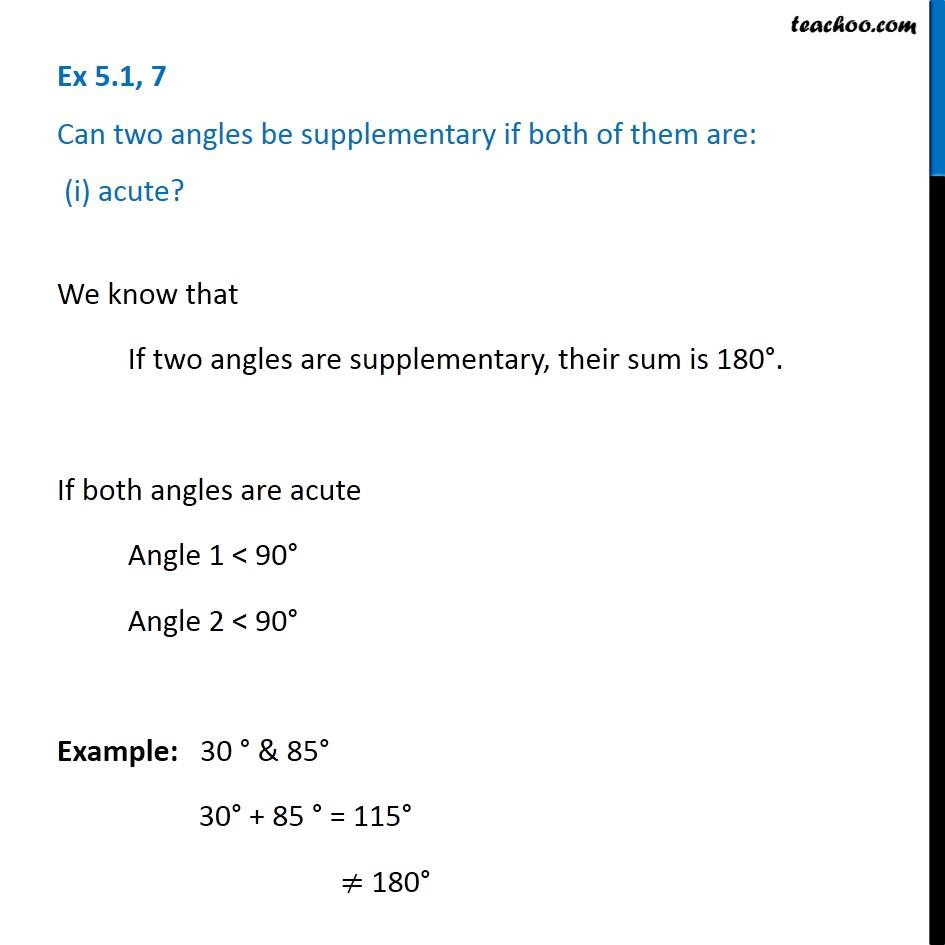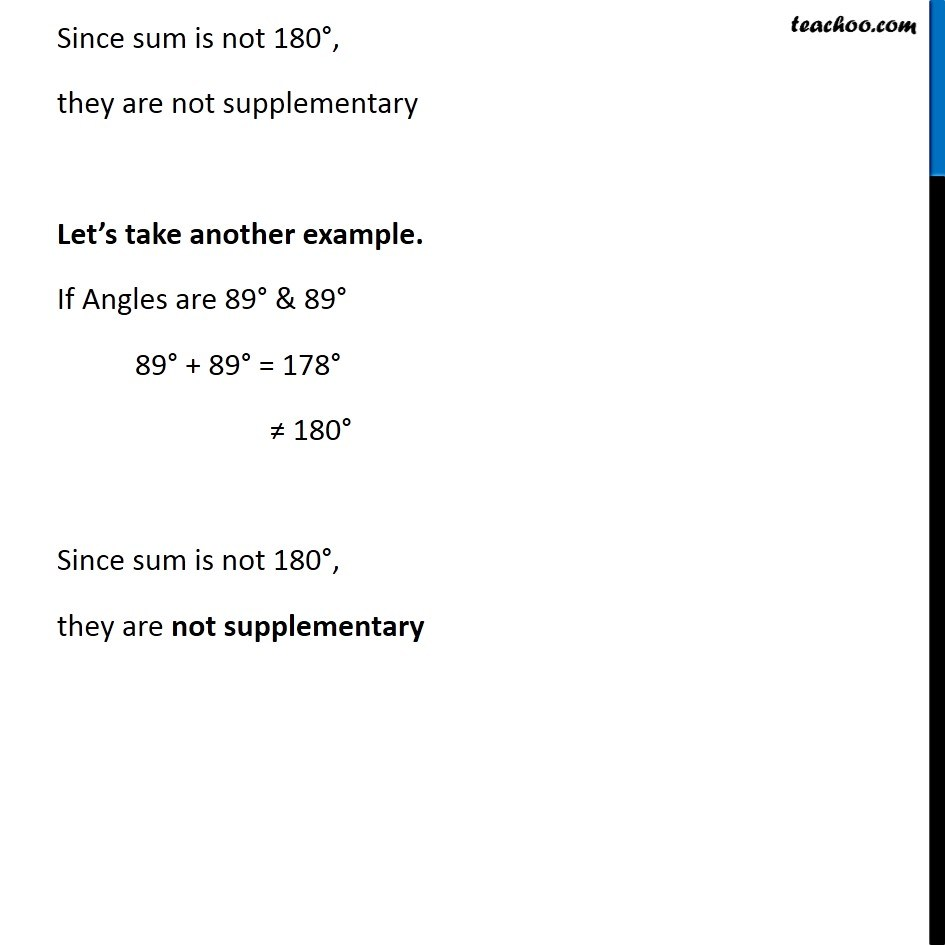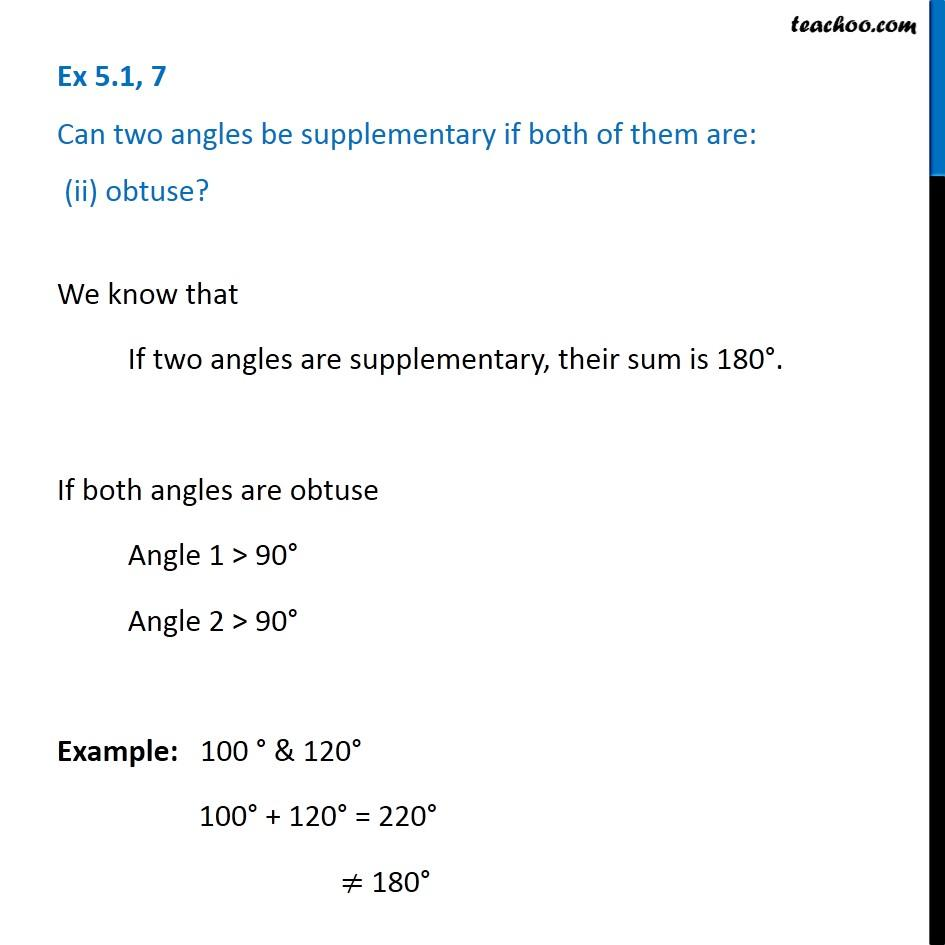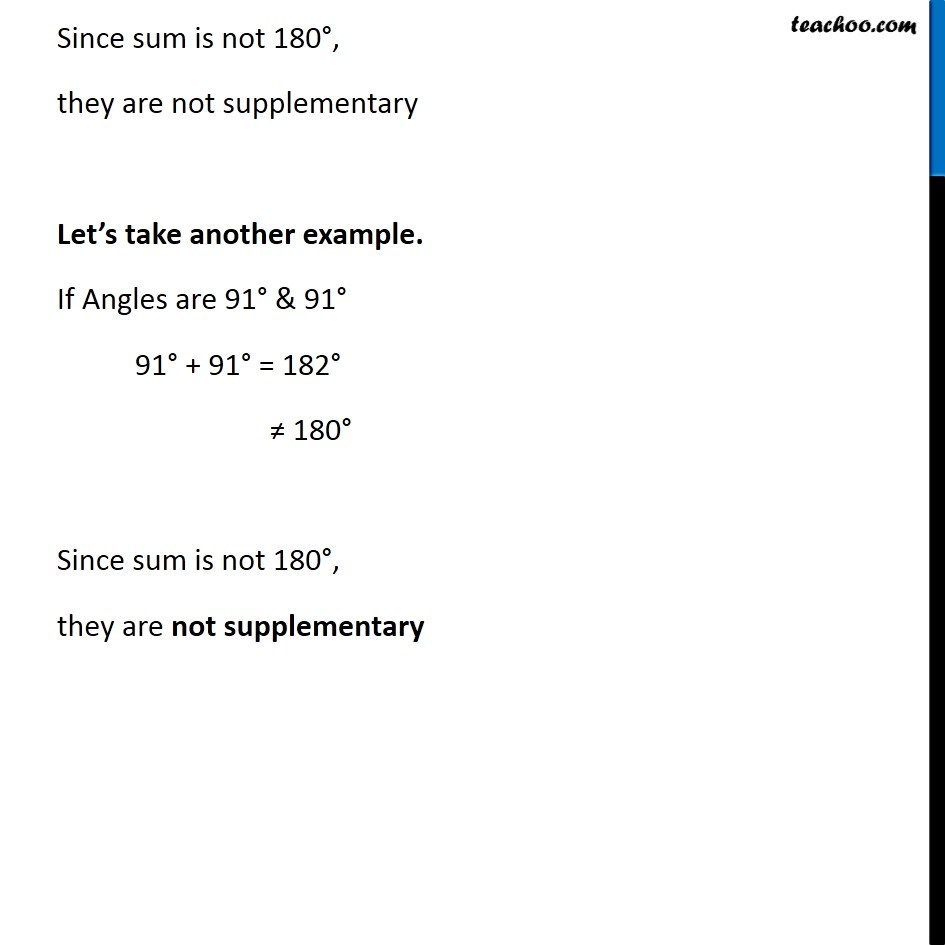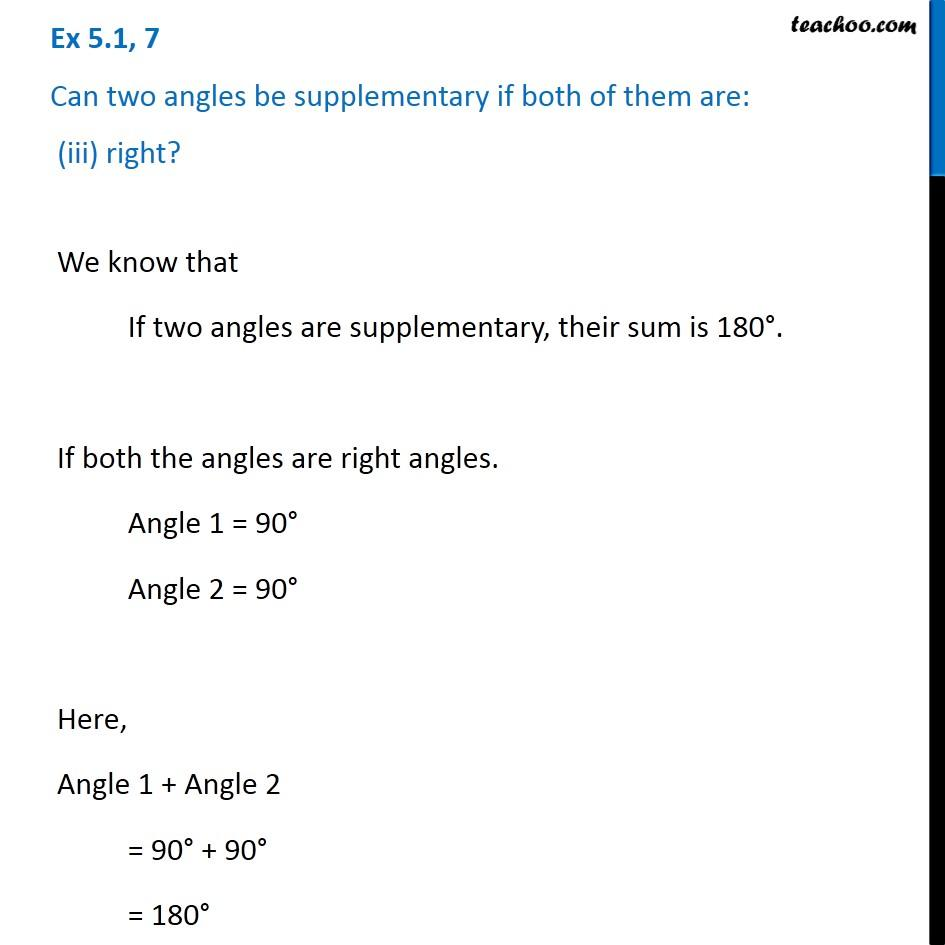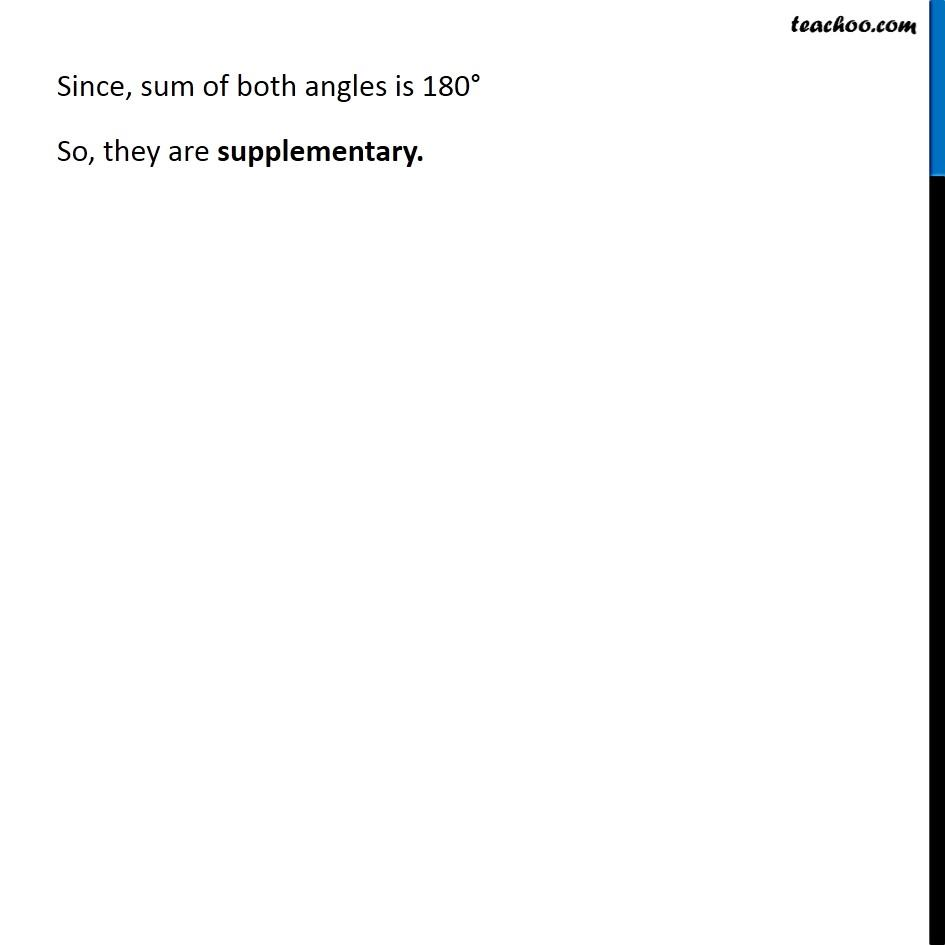Learn in your speed, with individual attention - Teachoo Maths 1-on-1 Class

### Transcript

Ex 5.1, 7 Can two angles be supplementary if both of them are: (i) acute? We know that If two angles are supplementary, their sum is 180°. If both angles are acute Angle 1 < 90° Angle 2 < 90° Example: 30 ° & 85° 30° + 85 ° = 115° ≠ 180° Since sum is not 180°, they are not supplementary Let’s take another example. If Angles are 89° & 89° 89° + 89° = 178° ≠ 180° Since sum is not 180°, they are not supplementary Ex 5.1, 7 Can two angles be supplementary if both of them are: (ii) obtuse? We know that If two angles are supplementary, their sum is 180°. If both angles are obtuse Angle 1 > 90° Angle 2 > 90° Example: 100 ° & 120° 100° + 120° = 220° ≠ 180° Since sum is not 180°, they are not supplementary Let’s take another example. If Angles are 91° & 91° 91° + 91° = 182° ≠ 180° Since sum is not 180°, they are not supplementary Ex 5.1, 7 Can two angles be supplementary if both of them are: (iii) right? We know that If two angles are supplementary, their sum is 180°. If both the angles are right angles. Angle 1 = 90° Angle 2 = 90° Here, Angle 1 + Angle 2 = 90° + 90° = 180° Since, sum of both angles is 180° So, they are supplementary.